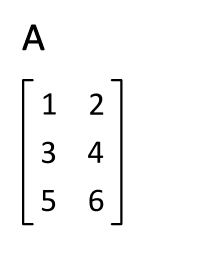V1

2022/09/20阅读：33主题：山吹

# R语言学习day4

## R语言矩阵

### 创建矩阵

R 语言的矩阵可以使用 `matrix()` 函数来创建，语法格式如下： `matrix(data = NA, nrow = 1, ncol = 1, byrow = FALSE,dimnames = NULL)`

• data 向量，矩阵的数据

• nrow 行数

• ncol 列数

• byrow 逻辑值，为 FALSE 按列排列，为 TRUE 按行排列

• dimname 设置行和列的名称，该参数用含有两个向量（字符串）的列表组成。

``> # 按行排列创建矩阵> zhen_1 = matrix(c(1:12),nrow = 3,byrow = TRUE)> print(zhen_1)     [,1] [,2] [,3] [,4][1,]    1    2    3    4[2,]    5    6    7    8[3,]    9   10   11   12> # 定义行和列的名称> rownames_1 = c("a1","a2","a3")> colnames_2 = c("b1","b2","b3","b4")> # 输出矩阵> out_1 = matrix(c(1:12),nrow = 3,byrow = TRUE,dimnames = list(rownames_1,colnames_2))> print(out_1)   b1 b2 b3 b4a1  1  2  3  4a2  5  6  7  8a3  9 10 11 12``

### 转置矩阵

R 语言矩阵提供了`t()`函数，可以实现矩阵的行列互换，变化效果如下：我们对刚刚创建的`out_1`矩阵进行转置后输出，如下：

``> out_1 = matrix(c(1:12),nrow = 3,byrow = TRUE,dimnames = list(rownames_1,colnames_2))> print(out_1)   b1 b2 b3 b4a1  1  2  3  4a2  5  6  7  8a3  9 10 11 12> print(t(out_1))  #输出转置矩阵用t（）   a1 a2 a3b1  1  5  9b2  2  6 10b3  3  7 11b4  4  8 12``

### 调用矩阵元素

``> print(out_1) #获取整个矩阵   b1 b2 b3 b4a1  1  2  3  4a2  5  6  7  8a3  9 10 11 12> print(out_1[1,2]) # 获取第1行第2列的元素 2> print(out_1[2,]) # 获取第2行的元素b1 b2 b3 b4  5  6  7  8 ``

### 矩阵的计算

``> # 矩阵相加，相减只需把加号改成减号> out_sum = m_1 + m_2  # 两个矩阵都是2×3> cat("结果：","\n")结果： > print(out_sum)     [,1] [,2] [,3][1,]   14   -2    4[2,]   18    8    6> # 矩阵相乘> out_cheng = m_1 * m_2  > cat("结果：","\n")结果： > print(out_cheng)     [,1] [,2] [,3][1,]   49    1    4[2,]   81   16    9> # 矩阵相除> out_chu = m_1 / m_2  > cat("结果：","\n")结果： > print(out_chu)     [,1] [,2] [,3][1,]    1    1    1[2,]    1    1    1``

## 数组

R 语言可以创建一维或多维数组，数组是一个同一类型的集合，矩阵就是一个二维数组。数组创建使用`array()`函数，该函数使用向量作为输入参数，可以使用 `dim` 设置数组维度。语法格式如下：

`array(data = NA, dim = length(data), dimnames = NULL)`

• data 向量，数组元素。
• dim 数组的维度，默认是一维数组。
• dimnames 维度的名称，必须是个列表

``> v1 = c(1,2,3)> v2 = c(4,5,6,7,8,9)> shuzu = array(c(v1,v2),dim = c(3,3,2))> print(shuzu), , 1     [,1] [,2] [,3][1,]    1    4    7[2,]    2    5    8[3,]    3    6    9, , 2     [,1] [,2] [,3][1,]    1    4    7[2,]    2    5    8[3,]    3    6    9``
• 设置各维度的名称
``> column.names = c("col1","col2","col3")  #设置列名> row.names = c("row1","row2","row3") #设置行名> matrix.names = c("m1","m2") #设置矩阵名> out_3 = array(c(v1,v2),dim = c(3,3,2),dimnames = list(row.names,column.names,matrix.names)) #设置数组名> print(out_3) #输出, , m1     col1 col2 col3row1    1    4    7row2    2    5    8row3    3    6    9, , m2     col1 col2 col3row1    1    4    7row2    2    5    8row3    3    6    9#上述m1和m2矩阵应用于笔记后面的演示，不再重复``
• 访问数组元素

``, , m2  #第二个矩阵     col1 col2 col3row1    1    4    7row2    2    5    8row3    3    6    9> print(out_3[3,1,2]) 3  #输出结果正确``
• 操作数组元素

``> matrix_1 = shuzu[,,1] > matrix_2 = shuzu[,,2]> sum_1 = matrix_1 + matrix_2> print(sum_1)     [,1] [,2] [,3][1,]    2    8   14[2,]    4   10   16[3,]    6   12   18``

`apply（）`函数能够对数组跨纬度计算，语法格式为：`apply(x, margin, fun)`

• x 数组
• margin 数据名称（1代表行，2代表列，c(1,2)代表行和列）
• fun 计算函数
``> print(shuzu)  , , 1   #数组的第一个矩阵     [,1] [,2] [,3][1,]    1    4    7[2,]    2    5    8[3,]    3    6    9, , 2  #数组的第二个矩阵     [,1] [,2] [,3][1,]    1    4    7[2,]    2    5    8[3,]    3    6    9> out_4 = apply(shuzu,1,sum)  #对数组所有矩阵按照每一行的方式求和> print(out_4) 24 30 36  #第一行数据24=1+4+7+1+4+7，确实能行！快速记忆：# 计算数组中所有矩阵对应行的数字之和result <- apply(shuzu, c(1), sum)# 计算数组中所有矩阵对应列的数字之和result <- apply(shuzu, c(2), sum)# 计算数组中每个矩阵内部的所有数字之和result <- apply(shuzu, c(3), sum)``V1